# Standard Deviations

The correct manipulation of standard deviations is an often overlooked skill. When working with data sets it is cruicial that the correct manipluations are applied for the results to be valid.

This page demonstrates several methods for combining standard deviations correctly with some worked examples.

## Addition and subtraction of values with standard deviations

Consider this problem where each value (a, b or c) has an associated standard deviation or error (Da, Db or Dc respectively). We are trying to calculate the correct value for z ± Dz: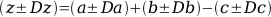z is easy to work out using conventional arithmetic: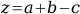whilst Dz can be calculated by using: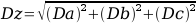Note that whether you add or subtract the raw values, the squares of the standard deviations are always added.

## Multiplication and division

### Multiplication and division by a constant

Multiplication and division are simpler when either multiplying or dividing by a constant value. In this case, simply multiply or divide the value and the standard deviation by the constant. Consider the problem: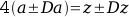In this case: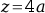and: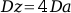### Multiplication and division by other uncertain values

Things are a little more complicated when multiplying or dividing by values that have their own associated uncertainties.

In this case, consider the problem: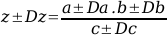z is worked out in the usual manner: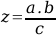However, when working out Dz the worst case scenario must be considered with all errors being added: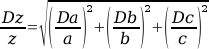First published on 21st September 2008 and last modified on 16th March 2011.